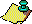# Glossary

In what follows we give a compact glossary of terms used in this paper.

1.   almost all A subset S of a set U contains `almost all' entries of U if its measure (in the sense of Lebesgue measure) is the same as that of U. A property that holds for almost all x in a set is said to hold `almost everywhere'. Probabilists also use the abbreviation a.s. for `almost surely', meaning with probability 1. ``Almost any reference on measure theory will give you more details.''---J. Borwein.

2.   asymptotically periodic orbits are orbits which eventually nearly repeat with a finite period.

3.   attractor An attractor of a map is a set of points which ``attracts'' orbits, from some set of initial points of nonzero probability of being selected. To be precise, an attractor of a map is an indecomposable closed invariant set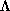with the property that, given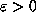, there is a set U of positive Lebesgue measure in the-neighbourhood ofsuch that if x is in U then the-limit set of orb(x) is contained inand the orbit of x is contained in U .
4.   basin of attraction The basin of attraction of an attractor is the set of initial points whose orbits tend to the attractor.

5.   chaos A dynamical system is chaotic if it is bounded and sensitive to initial conditions. Both conditions are needed, because a simple linear map such as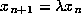has positive Lyapunov exponent and is thus sensitive to initial conditions but is not chaotic, while bounded orbits that don't diverge from one another are too regular to be called `chaotic'. There is a more mathematically flavoured definition, usually attributed to Devaney, which uses transitivity. This is, to my mind, not the essence of chaos, though it allows precise discussion.
6.   discrete dynamical system A smooth invertible function f from a set B to itself, which we iterate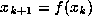. In this paper we use the term loosely, forgetting about the smoothness and invertibility (see map).

7.   dimension Sets have dimensions, which can be defined in various ways. The most popular mathematical dimension is the Hausdorff dimension, which is often difficult to compute, but is well-defined and interesting. Other dimensions of interest include the capacity dimension (also called the `box-counting' dimension), the correlation dimension, and the information dimension. When non-integer in value, any of these are loosely referred to as the `fractal' dimension. See e.g.  for more details.

8.   Euclidean Algorithm Suppose we are given two integers a and b. Without loss of generality assume that both are positive and that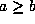. Put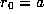and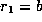. Define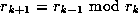, for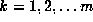where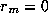terminates the process. Then the gcd of a and b is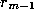. For more details, and to investigate the connection to continued fractions, see . For a deeper discussion see .

9.   integer part In converting a real number to an integer, we can round towards infinity (the ceiling function), towards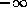(the floor function), towards 0 (the truncate function), or towards the nearest integer (the round function). By `integer part' I mean the `floor' function, so that the fractional part is always nonnegative.
10.   fixed points Any points x that satisfy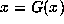are called fixed points of the map.

11.   fixed precision arithmetic Another name for floating-point arithmetic.

12.   floating-point arithmetic The usual style of computer arithmetic, familiar to most of us at least from calculators. There are several variants, but the most important for our purposes are IEEE standard floating-point arithmetic and the arbitrary-precision floating-point arithmetic found in most computer algebra systems. The main advantages of floating-point arithmetic are speed and predictable memory usage, and the main disadvantage is that it must be inexact, because numbers must be mutilated in order to fit into the fixed storage allocated for them.
13.   fractional part The fractional part of x is x - floor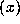.

14.   gcd Greatest Common Divisor. The gcd of two integers a and b is the largest positive integer d such that d divides into a and into b evenly. It can be computed by the Euclidean Algorithm.

15.   indecomposable set An invariant set is indecomposable with respect to a map G if it cannot be partitioned into mutually exclusive invariant subsets.

16.   invariant set An invariant set is a set such that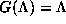.

17.   limit set The-limit set of orb(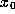) is the set of all initial points whose orbits approach orb() as ``time'' increases; to be precise, an initial point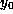is in the-limit set of orb() if there exist m and n such that for allthere exists K such that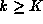implies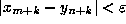. The-limit set of orb() is the set of accumulation points of orb().

18.   Lyapunov exponents Given a differentiable map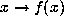, we can look at the induced map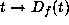in the tangent space, formed by computing the Jacobian matrix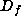of f. We can then look at how a unit ball in the tangent space atevolves under iteration of this tangent space map, which measures in some sense the growth of small initial errors as the map is iterated. We can then look at the `average' rate of growth of the images of this unit ball, by considering the SVD of the product of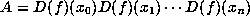(equivalently, the square roots of the eigenvalues of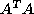). The limiting exponential rate of growth of these singular values (which Osledec  showed existed for almost all initial points, for regular dynamical systems f) are called the Lyapunov exponents of the map. In our one-dimensional case, this amounts to computing the limit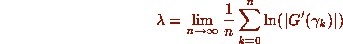where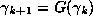gives the orbit of G starting at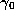. That is the definition, but it doesn't really tell you what the Lyapunov exponents are good for. Click here for a tutorial that will explore Lyapunov exponents further.

19.   map A function f from a set B to itself, which we iterateto define an orbit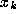,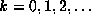.

20.   measure-preserving transformation We say that the transformation (or map) f preserves the measure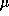if the measure of a set A is the same as the sum of the measures of all the pre-images of A under f: that is,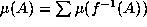. Since f may be many-to-one, this is a different definition than would result if we asked for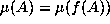.

For the Gauss map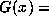frac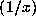, we can fairly easily show that it preserves the Gauss measure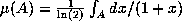by considering the so-called basic sets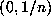for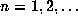. The pre-image of the setis the set of intervals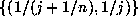for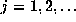. The measures of these intervals add up to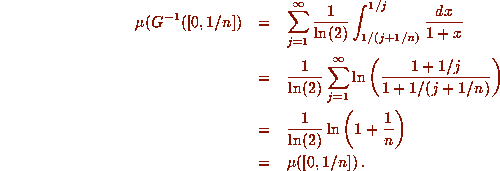Other sets in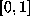may be formed by countable unions and intersections of these sets, and hence the Gauss measure is preserved by the Gauss map. The only difficult part of this computation is the evaluation of the sum in the middle, and this is tedious but straightforward. In passing I note that Maple can evaluate this sum, when properly coached.
21.   noble numbers A number is called `noble' if its continued fraction is ultimately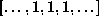.

22.   orbit The set of iteratesof a (here discrete) dynamical system. Strictly speaking, we allow negative k (because f is invertible), but in this paper I loosely refer to a `forward' orbit, with nonnegative k only, simply as an `orbit'. We denote this by orb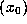.

23.   periodic points If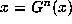where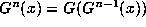then x is called a periodic point of the map. If N is the least such number n, then as usual we say x has period N.

24.   quadratic irrational A root of a quadratic polynomial with integer coefficients.

25.   reconstruction A process by which one tries to recover a continuous dynamical system from discrete samples of its output. There is a large literature on the subject. See  for details.

26.   reduced quadratic irrational A quadratic irrationalwhose conjugate (i.e. the other root of the quadratic polynomial with integer coefficients thatsatisfies) lies in the interval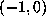.

27.   Sensitive to Initial Conditions, or S.I.C. A map G is said to be sensitive to initial conditions (SIC) if initially close initial points have orbits that separate at an exponential rate. Two orbits separate at an exponential rate if their differences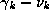grow like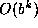for some base b, at least initially. Another, more rigorous, definition of this is that the Lyapunov exponent of one or both orbits is positive. Still another definition is that points that are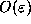close initially will, after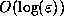iterations of the map, be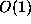apart.

28.   shift map Given a finite list of symbols (e.g. 0, 1, and 2 or A, B, C, .. Z) we can consider sequences of such symbols, either semi-infinite (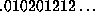) or bi-infinite (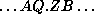, with a reference point in the middle). The `shift map' is a map which moves each entry in the sequence one entry to the left: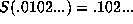. See  for discussion and application.
29.   simple continued fraction A fraction of the form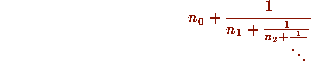where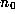is an integer and all the other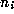are positive integers. See  for more details. Theare called the partial quotients.

30.   simulation Computing orbits with floating-point arithmetic. Some authors use `discretization' for this (e.g. Diamond) but I reserve that word for the process of converting a continuous dynamical system to a discrete one, perhaps by approximation by finite differences.

31.   ultimately periodic An orbit is ultimately periodic if there exists an integer K (the length of the `transient') and another integer P (the `period') such that for all k > K we have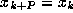.